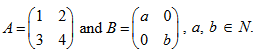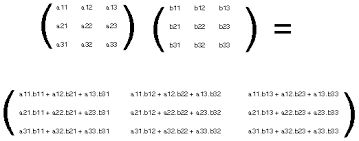#### LetThen Option 1) there cannot exist any B such that AB = BA Option 2) there exist more than one but finite number B's such that AB = BA Option 3) there exists exactly one B such that AB = BA Option 4) there exist infinitely many B's such that AB = BA

As we learnt in

Multiplication of matrices --2b = 2a

3a  = 3bOption 1)

there cannot exist any B such that AB = BA

Incorrect Option

Option 2)

there exist more than one but finite number B's such that AB = BA

Incorrect Option

Option 3)

there exists exactly one B such that AB = BA

Incorrect Option

Option 4)

there exist infinitely many B's such that AB = BA

Correct Option

#### Vakul